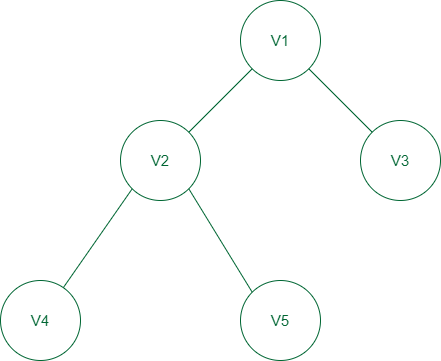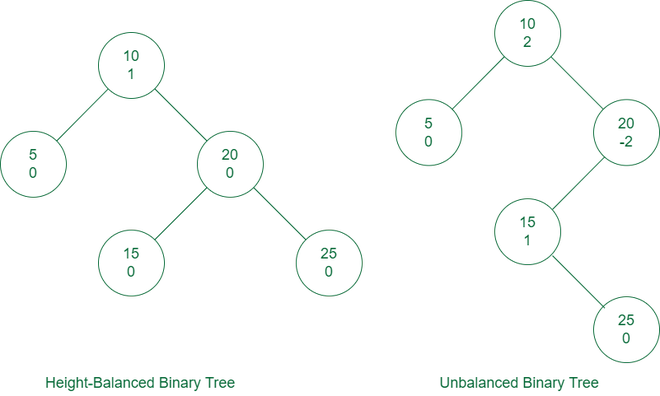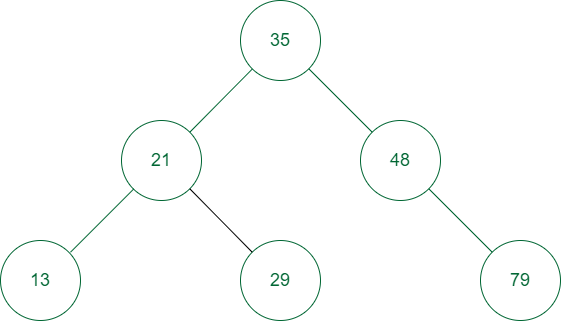# Introduction to Height Balanced Binary Tree

• Last Updated : 19 May, 2022

A height-balanced binary tree is defined as a binary tree in which the height of the left and the right subtree of any node differ by not more than 1. AVL tree, red-black tree are examples of height-balanced trees.Height Balanced tree

## Conditions for Height-Balanced Binary Tree:

Following are the conditions for a height-balanced binary tree:

• The difference between the heights of the left and the right subtree for any node is not more than one.
• The left subtree is balanced.
• The right subtree is balanced.

Note: An empty tree is also height-balanced.

## What is the Height Balance of a node?

To check if the binary tree is height-balanced or not, you have to check the height balance of each node. For this, you need to calculate the heights of the two subtrees for each node making this impractical. Instead, we store the height balance information of every subtree in the root node of that subtree. Thus, each node not only maintains its data and children’s information but also a height balance value.
The height balance of a node is calculated as follows:

height balance of node  = height of right subtree – height of left subtree

The above formula means that:

• If the right subtree is taller, the height balance of the node will be positive
• If the left subtree is taller, the balance of the node will be negative.Height-balanced and Unbalanced binary tree

## Height of a Height-Balanced Binary Tree:

The height of a node in a tree is the length of the longest path from that node downward to a leaf, counting both the start and end vertices of the path. The height of a leaf is 1. The height of a nonempty tree is the height of its root. It can be proved that the height of a height-balanced binary tree with N nodes is O(logN).

Proof:

The minimum number of nodes in a height-balanced binary tree of height h is greater than 2h/2-1 nodes and let this is denoted by the function f(h), i.e. f(h) > 2h/2-1

This can be proved using mathematical induction.

• A height-balanced binary tree of height 1 has at least 2 node. So f(1) = 2 > 21/2 – 1 .
• A height-balanced binary tree of height 2 has a minimum of 4 nodes i.e., the root node, its two children and at least one node at depth of 2. So f(2) = 4 > 22/2 – 1.
• Now consider the statement is true for some value of height (h-1) > 2. So we have to prove that it is also valid for height = h.
A tree of height h, has a root and its two subtrees. One of the subtrees will have height h-2 and the other h-1 (because we want the minimum number of nodes). So,
f(h) = 1 + f(h-1) + f(h-2)
f(h) > 1 + 2 * f(h-2)  because f(h-1) > f(h-2)
f(h) > 2 * f(h-2).
As the statement is true for values less than h,
So f(h) > 2*2(h-2)/2 – 1
i.e., f(h) > 2h/2-1.
• So we can see
log( f(h) ) > h/2 – 1 or
h/2 < log( f(h) ) + 1
h < 2*log( f(h) ) + 2
h < 2*log(N) + 2    [say the minimum number of nodes is N. So f(h) = N]

Therefore it is proved that h = O(logN)

## Why do we need a Height-Balanced Binary Tree?

Let’s understand the need for a balanced binary tree through an example.Binary search tree

The above tree is a binary search tree and also a height-balanced tree.
Suppose we want to want to find the value 79 in the above tree. First, we compare the value of the root node. Since the value of 79 is greater than 35, we move to its right child, i.e., 48. Since the value 79 is greater than 48, so we move to the right child of 48. The value of the right child of node 48 is 79. The number of hops required to search the element 79 is 2.
Similarly, any element can be found with at most 2 jumps because the height of the tree is 2.

So it can be seen that any value in a balanced binary tree can be searched in O(logN) time where N is the number of nodes in the tree. But if the tree is not height-balanced then in the worst case, a search operation can take O(N) time.

## Applications of Height-Balanced Binary Tree:

• Balanced trees are mostly used for in-memory sorts of sets and dictionaries.
• Balanced trees are also used extensively in database applications in which insertions and deletions are fewer but there are frequent lookups for data required.
• It is used in applications that require improved searching apart from database applications.
• It has applications in storyline games as well.
• It is used mainly in corporate sectors where they have to keep the information about the employees working there and their change in shifts.

## Advantages of Height-Balanced Binary Tree:

• It will improve the worst-case lookup time at the expense of making a typical case roughly one lookup less.
• As a general rule, a height-balanced tree would work better when the request frequencies across the data set are more evenly spread,
• It gives better search time complexity.

## Disadvantages of Height-Balanced Binary Tree:

• Longer running times for the insert and remove operations.
• Must keep balancing info in each node.
• To find nodes to balance, must go back up in the tree.

## How to check if a given tree is height-balanced:

You can check if a tree is height-balanced using recursion based on the idea that every subtree of the tree will also be height-balanced. To check if a tree is height-balanced perform the following operations:

• Use recursion and visit the left subtree and right subtree of each node:
• Check the height of the left subtree and right subtree.
• If the absolute difference between their heights is at most 1 then that node is height-balanced.
• Otherwise, that node and the whole tree is not balanced.

Refer to our article on “How to determine if a binary tree is height-balanced” for implementation of this.

My Personal Notes arrow_drop_up
Recommended Articles
Page :